# User:Jjtorrens/BancoDePruebas

(diff) ← Older revision | Latest revision (diff) | Newer revision → (diff)

2010 Mathematics Subject Classification: Primary: 46E35 [MSN][ZBL]

$\newcommand{\abs}{\lvert #1\rvert} \newcommand{\norm}{\lVert #1\rVert} \newcommand{\bfl}{\mathbf{l}}$ A locally summable generalized derivative of a locally summable function (see Generalized function).

More explicitly, ifis an open set in an-dimensional spaceand ifandare locally summable functions on, thenis the Sobolev generalized partial derivative with respect to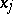ofon: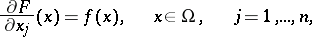if the following equation holds: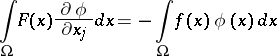for all infinitely-differentiable functionsonwith compact support. The Sobolev generalized derivative is only defined almost-everywhere on.

An equivalent definition is as follows: Suppose that a locally summable functiononcan be modified in such a way that, on a set of-dimensional measure zero, it will be locally absolutely continuous with respect tofor almost-all points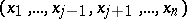, in the sense of the-dimensional measure. Thenhas an ordinary partial derivative with respect tofor almost-all. If the latter is locally summable, then it is called a Sobolev generalized derivative.

A third equivalent definition is as follows: Given two functionsand, suppose there is a sequenceof continuously-differentiable functions onsuch that for any domainwhose closure lies in,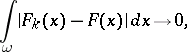Thenis the Sobolev generalized derivative ofon.

Sobolev generalized derivatives ofonof higher orders (if they exist) are defined inductively: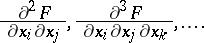They do not depend on the order of differentiation; e.g.,almost-everywhere on.

How to Cite This Entry:
Jjtorrens/BancoDePruebas. Encyclopedia of Mathematics. URL: http://encyclopediaofmath.org/index.php?title=Jjtorrens/BancoDePruebas&oldid=26003